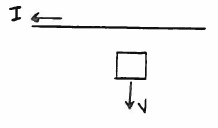# Problem: A long straight wire carries constant current I that is directed toward the left, as shown in the sketch. A small conducting loop is moving away from the wire with constant speed v. The current induced in the loop is A) zero B) clockwise C) counterclockwise

🤓 Based on our data, we think this question is relevant for Professor Voronine's class at USF.

###### Problem Details

A long straight wire carries constant current that is directed toward the left, as shown in the sketch. A small conducting loop is moving away from the wire with constant speed v. The current induced in the loop is

A) zero

B) clockwise

C) counterclockwiseWhat scientific concept do you need to know in order to solve this problem?

Our tutors have indicated that to solve this problem you will need to apply the Lenz's Law concept. You can view video lessons to learn Lenz's Law. Or if you need more Lenz's Law practice, you can also practice Lenz's Law practice problems.

What is the difficulty of this problem?

Our tutors rated the difficulty ofA long straight wire carries constant current I that is dire...as medium difficulty.

How long does this problem take to solve?

Our expert Physics tutor, Juan took 4 minutes and 24 seconds to solve this problem. You can follow their steps in the video explanation above.

What professor is this problem relevant for?

Based on our data, we think this problem is relevant for Professor Voronine's class at USF.